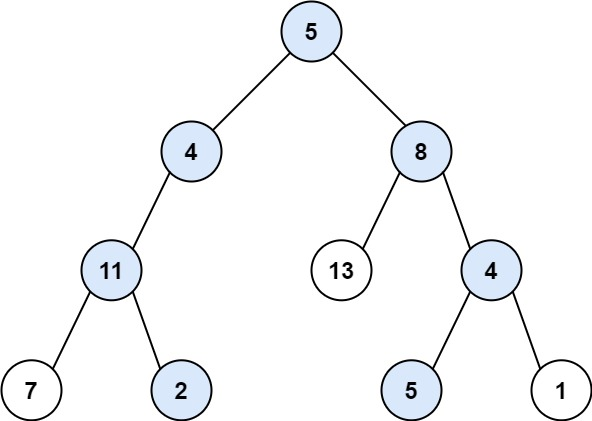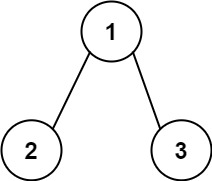## C# || How To Get All Root To Leaf Binary Tree Paths Equal To Path Sum Using C#The following is a module with functions which demonstrates how to get all the root to leaf binary tree paths equal to path sum using C#.

1. Root To Leaf Path Sum – Problem Statement

Given the root of a binary tree and an integer targetSum, return all root-to-leaf paths where the sum of the node values in the path equals targetSum. Each path should be returned as a list of the node values, not node references.

A root-to-leaf path is a path starting from the root and ending at any leaf node. A leaf is a node with no children.

Example 1:``` Input: root = [5,4,8,11,null,13,4,7,2,null,null,5,1], targetSum = 22 Output: [[5,4,11,2],[5,8,4,5]] Explanation: There are two paths whose sum equals targetSum: 5 + 4 + 11 + 2 = 22 5 + 8 + 4 + 5 = 22 ```

Example 2:``` Input: root = [1,2,3], targetSum = 5 Output: [] ```

Example 3:

``` Input: root = [1,2], targetSum = 0 Output: [] ```

2. Root To Leaf Path Sum – Solution

The following is a solution which demonstrates how to get all the root to leaf binary tree paths equal to path sum.

The main idea here is that the sum at each level for each path is calculated until we reach the end of the root-to-leaf.

A list is used to store the node value at each level. When the next level is explored, the value is appended to the list, and the process continues.

When we reach the end of the leaf, we check to see if the target value has been reached. If so, we add the node values that make up the path to the result list.

``` 2. Root To Leaf Path Sum - Solution C# // ============================================================================ // Author: Kenneth Perkins // Date: Oct 18, 2021 // Taken From: http://programmingnotes.org/ // File: Solution.cs // Description: Determines how to get all root to leaf path sum in a tree // ============================================================================ /** * Definition for a binary tree node. * public class TreeNode { * public int val; * public TreeNode left; * public TreeNode right; * public TreeNode(int val=0, TreeNode left=null, TreeNode right=null) { * this.val = val; * this.left = left; * this.right = right; * } * } */ public class Solution { private List<IList<int>> result = new List<IList<int>>(); public IList<IList<int>> PathSum(TreeNode root, int targetSum) { Search(root, targetSum, 0, new List<int>()); return result; } private void Search(TreeNode node, int targetSum, int currentSum, List<int> path) { if (node == null) { return; } // Add the node to this path path.Add(node.val); // Add the current value to the running total currentSum = currentSum + node.val; // Since this is a root-to-leaf check, evaluate for the // success condition when both left and right nodes are null if (node.left == null && node.right == null) { // Check to see if current value equals target if (currentSum == targetSum) { result.Add(new List<int>(path)); } } // Keep exploring along branches finding the target sum Search(node.left, targetSum, currentSum, path); Search(node.right, targetSum, currentSum, path); // Remove the last item added as this path has already been explored path.RemoveAt(path.Count - 1); } }// http://programmingnotes.org/ 123456789101112131415161718192021222324252627282930313233343536373839404142434445464748495051525354555657 // ============================================================================//    Author: Kenneth Perkins//    Date:   Oct 18, 2021//    Taken From: http://programmingnotes.org///    File:  Solution.cs//    Description: Determines how to get all root to leaf path sum in a tree// ============================================================================/** * Definition for a binary tree node. * public class TreeNode { *     public int val; *     public TreeNode left; *     public TreeNode right; *     public TreeNode(int val=0, TreeNode left=null, TreeNode right=null) { *         this.val = val; *         this.left = left; *         this.right = right; *     } * } */public class Solution {    private List<IList<int>> result = new List<IList<int>>();     public IList<IList<int>> PathSum(TreeNode root, int targetSum) {        Search(root, targetSum, 0, new List<int>());        return result;    }     private void Search(TreeNode node, int targetSum, int currentSum, List<int> path) {        if (node == null) {            return;        }         // Add the node to this path        path.Add(node.val);         // Add the current value to the running total        currentSum = currentSum + node.val;         // Since this is a root-to-leaf check, evaluate for the        // success condition when both left and right nodes are null        if (node.left == null && node.right == null) {             // Check to see if current value equals target            if (currentSum == targetSum) {                result.Add(new List<int>(path));            }        }         // Keep exploring along branches finding the target sum        Search(node.left, targetSum, currentSum, path);        Search(node.right, targetSum, currentSum, path);         // Remove the last item added as this path has already been explored        path.RemoveAt(path.Count - 1);    }}// http://programmingnotes.org/ ```

QUICK NOTES:
The highlighted lines are sections of interest to look out for.

The code is heavily commented, so no further insight is necessary. If you have any questions, feel free to leave a comment below.

Once compiled, you should get this as your output for the example cases:

``` [[5,4,11,2],[5,8,4,5]] [] [] ```